# Algebra 1 : Functions and Lines

## Example Questions

1 2 87 88 89 90 91 92 93 95 Next →

### Example Question #941 : Functions And Lines

Find the inverse of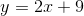.

Possible Answers: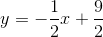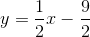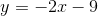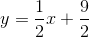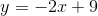Correct answer:Explanation:

Interchange the x and y variables.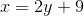Subtract nine from both sides.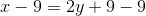Simplify both sides.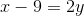Divide by two on both sides.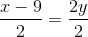Simplify the fractions.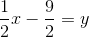The inverse is:### Example Question #25 : How To Find Inverse Variation

The cost to go on a field trip varies inversely with the number of people attending the trip. If it costs $10 to go on the trip when 3 people are attending, how much would it cost if 15 people are attending? Possible Answers:$8

$50$5

$2 Correct answer:$2

Explanation:

Because the cost varies inversely with the number of people attending, the formula is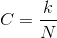Whereis the cost andis the number of people attending.

We first solve forby plugging in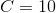and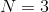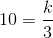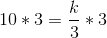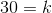We then evaluatewhen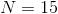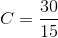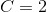Therefore the cost is

\$2

### Example Question #26 : How To Find Inverse Variation

Find the inverse: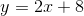Possible Answers: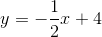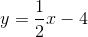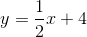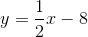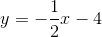Correct answer:Explanation:

Interchange the x and y variables.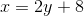Solve for y.  Subtract eight on both sides.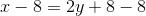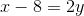Divide by two on both sides.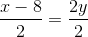Simplify both sides.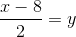The answer is:### Example Question #27 : How To Find Inverse Variation

Find the inverse of: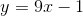Possible Answers: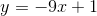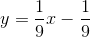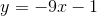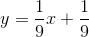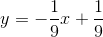Correct answer:Explanation:

Interchange the x and y variables.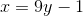Solve for y.   Add 1 on both sides and simplify.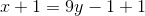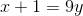Divide by nine on both sides.

The answer is:### Example Question #28 : How To Find Inverse Variation

Find the inverse of: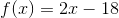Possible Answers: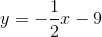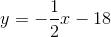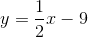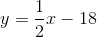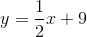Correct answer:Explanation:

Replace theterm with.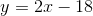Interchange theandvariables.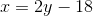Solve for. Addon both sides.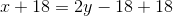Simplify both sides.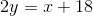Divide by two on both sides.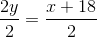Simplify both sides.

The answer is:### Example Question #941 : Functions And Lines

Find the inverse of: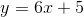Possible Answers: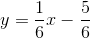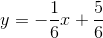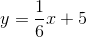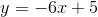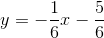Correct answer:Explanation:

Interchange the x and y-variables.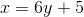Solve for y.  Subtract five from both sides.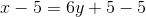Simplify the right side.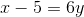Divide by six on both sides.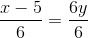Simplify both sides.

The answer is:### Example Question #942 : Functions And Lines

Find the inverse of: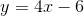Possible Answers: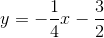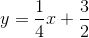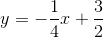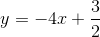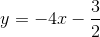Correct answer:Explanation:

To find the inverse, interchange the x and y-variables.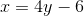Solve for y.   Add 6 on both sides of the equation.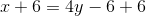Simplify the right side.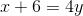Divide by four on both sides.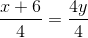Simplify both sides.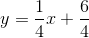Simplify the y-intercept.

The answer is:1 2 87 88 89 90 91 92 93 95 Next →

### All Algebra 1 Resources